# Statistical tools

### Play with our tools to understand some statistical concepts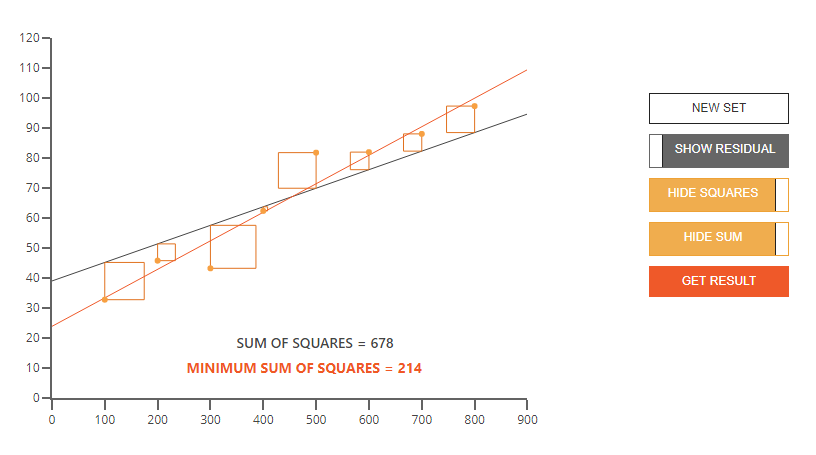### Least Squares Regression

Users try to find best fit line guided by squared errors and the Residual Sum of Square.

Launch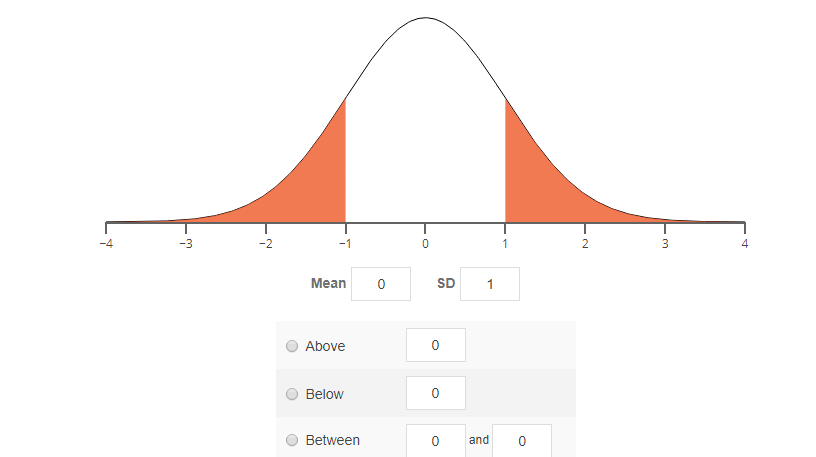### Calculate a probability

How to compute the probabilities from the Normal lay and understand the importance for statistics.

Launch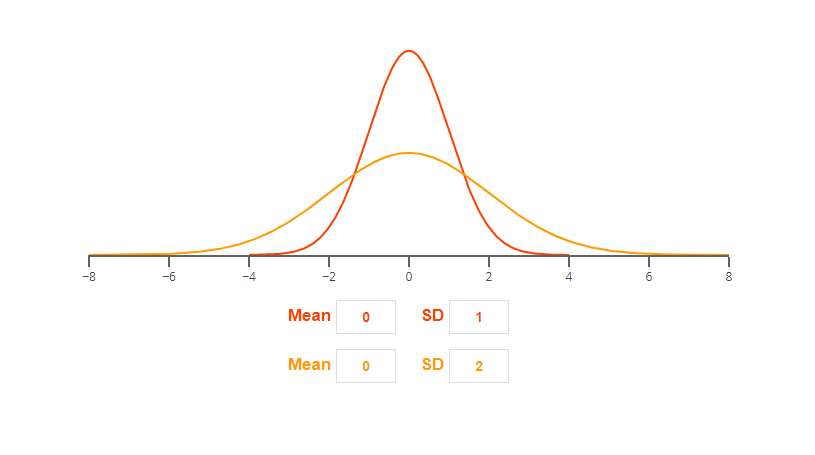### Central point and dispersion

Impact of the mean and of the standard deviation on the Normal distribution.

Launch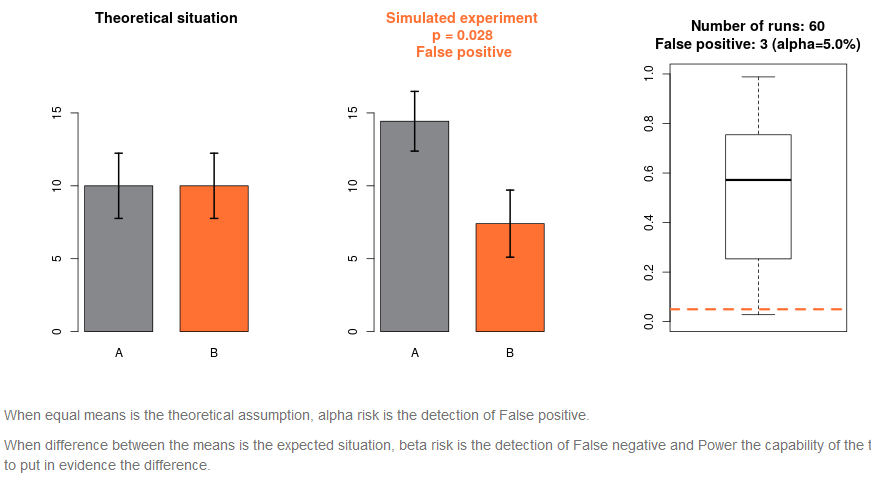### Alpha, Power and Sample Size

Understand the statistical risks, alpha-type and beta-type (power), through the Student’s T-Test.

Launch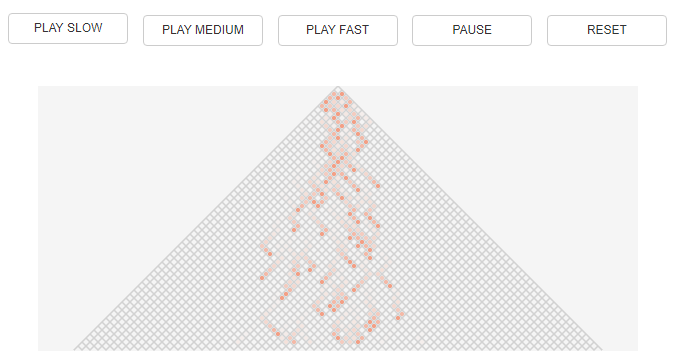### Central Limit Theorem

Build the Gaussian distribution with successive series of coin game.

Launch